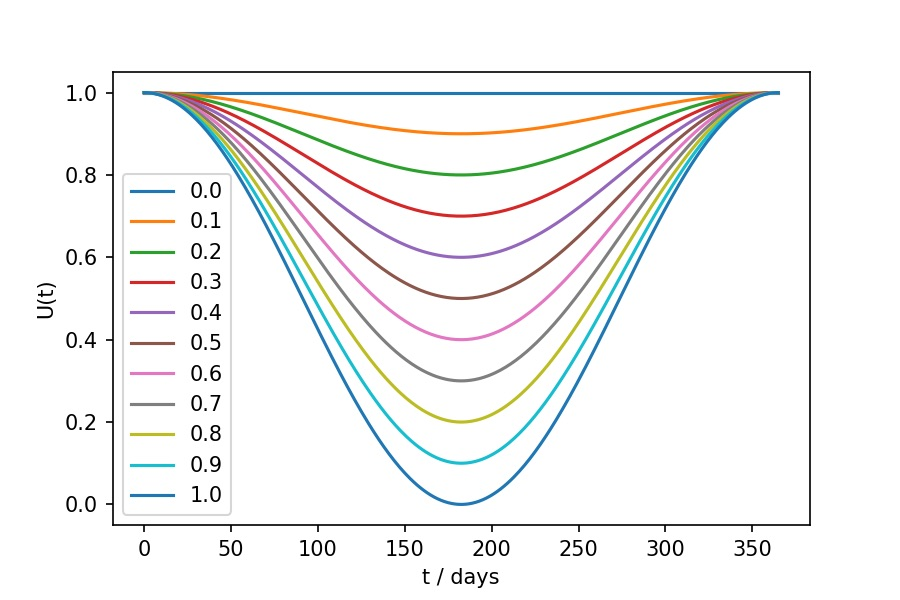# Alternative lockdowns

The flexibility of metawards means that there are multiple different ways you could choose to model a lockdown.

To understand how, we must look at how metawards calculates the force of infection (FOI) of each ward. The FOI of a ward is used to calculate the infection rate, which determines the rate by which individuals in a ward become infected.

## Force of infection

The force of infection (FOI) is calculated for each ward individually, using the equation;

1. $$F(w) = S \times U(t) \times \sum_s [ C_s \beta_s N_s(w) ]$$

where;

• $$F(w)$$ is the force of infection, $$F$$, calculated for a specific ward, $$w$$,

• $$S$$ is a constant scaling parameter, set via Population.scale_uv,

• $$U(t)$$ is a seasonal scaling function (UV) calculated for the specified day $$t$$,

• $$\sum_s$$ is the sum over all disease stages, $$s$$,

• $$C_s$$ is the contrib_foi disease parameter for stage $$s$$,

• $$\beta_s$$ is the beta disease parameter for stage $$s$$, and

• $$N_s(w)$$ is the number of infected individuals in ward $$w$$ in disease stage $$s$$.

The seasonal scaling function, $$U(t)$$ is calculated via;

1. $$U(t) = 1.0 - \frac{U_v}{2.0} + U_v \frac{\text{cos}(2 \pi t / 365)}{2.0}$$

where;

• $$U_v$$ is the UV parameter supplied by the user --UV command line argument, and

• $$t$$ is the number of days between the current date of the model run and the date at which seasonal spread is at a maximum, UV_max, set via the user --UV-max command line argument.

The seasonal scaling function acts to scale down the force of infection from a seasonal maximum (typically 1st January to model winter diseases in the northern hemisphere) to a seasonal minimum 6 months later. $$U_v$$ should be set to between 0 and 1, and is the scaling factor at the six month minimum. The $$U(t)$$ functions for different values of $$U_v$$ are shown below;## Metapopulation movements

The force of infection calculation is based on the number of infected individuals at each disease stage in each ward, $$N_s(w)$$. The metapopulation part of metawards is because individuals move between wards, and thus will contribute to $$N_s(w)$$ differently depending on when and where they are.

The force of infection is calculated both for daytime and nighttime. Individuals contribute to the force of infection calculation for wards they visit during the daytime, and to their home ward at nighttime.

Workers visit their fixed work ward during the daytime, as long as they are not too sick to go to work (controlled by too_sick_to_move, and in which case they stay at home). Players will visit a randomly chosen play ward during the daytime.

However, these movements are controlled by a cutoff parameter, Parameters.dyn_dist_cutoff. If the distance between the home and work ward, or home and play ward, is greater than the cutoff (measured in kilometers), then the worker or player will stay at home, and contribute to the force of infection of their home ward.

Note

This is all calculated in the advance_foi() advance function. If you want to change this, you can write your own version of advance_foi() and set that to be used instead via a custom iterator.

## Rates of infection

The daytime and nighttime forces of infection for each ward are converted into daytime and nighttime infection probabilities by the advance_infprob() advance function. These give the probability that an individual staying in the ward that day or night would be infected. These probablities are used by the advance_fixed() and advance_play() advance functions to determine whether workers or players, as they move between wards each day, would be infected.

## Enacting lockdown

Based on this knowledge, we could enact a lockdown by adjusting the parameters used to calculate the force of infection, and the parameters used to control movement of individuals between wards. For example, we could reduce Population.scale_uv, thereby reducing $$S$$ in equation 1. This would have the same effect on scaling down the FOI as scaling down $$\beta$$. This would mean that you could relate different values of $$S$$ to different lockdown control measures, e.g. closing schools, wearing masks, limiting number of contacts etc.

We could also reduce Parameters.dyn_dist_cutoff to, e.g. 5 km, to prevent most work and play movements. Indeed, we could even reduce this to 0 km to stop all movement between wards.

A good example of an alternative lockdown model is here. This is provided as an example in the MetaWards GitHub repository, and enacts lockdown by directly changing these two parameters. This has the effect of reducing the contribution from each infected individual to the overall force of infection of each ward, and reducing the movement of individuals between wards.

There are many parameters to adjust. You can also add these to your scan to investigate their impact. The full list of built-in adjustable parameters is below;

UV:

UV_max:

beta:

bg_foi:

contrib_foi:

daily_imports:

dyn_dist_cutoff:

dyn_play_at_home:

initial_inf:

length_day:

play_to_work:

progress:

scale_uv:

static_play_at_home: